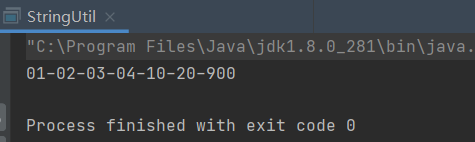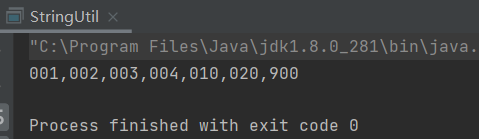• 整形转字符串
2019-06-25 16:45:12

项目中要用到文件操作输出一些结构体信息，包括一些int型的数字，为了直观，需要转换成字符串，虽然c语言提供了这种函数，但还是想自己从底层造个轮子。思路：把int的位数记住，申请位数加1的内存(多一个存'\0')。把每一个数字转换成字符存入。

// 整型转字符串.cpp : 此文件包含 "main" 函数。程序执行将在此处开始并结束。
//

#include "pch.h"
#include <iostream>
using namespace std;

static void int_change_str(int num,char **str)
{
if (num == 0) //num为0 直接返回
{
*str = (char*)malloc(sizeof(char)+1);
**str = '0' + 0;
(*str) = '\0';
//printf("%s\n", *str);
return;
}
int weishu = 0;//记录num有多少位
char *temp = (char*)malloc(sizeof(char)*100);//倒序暂存 100字节
while (num!=0)
{
temp[weishu] = '0' + num % 10;
num /= 10;
weishu++;

}
*str = (char*)malloc(sizeof(char)*weishu + 1);
for (int i = 0; i < weishu; i++)
{
(*str)[i] = temp[weishu - i - 1];
}
(*str)[weishu] = '\0';
//printf("%s\n", *str);
}

int main()
{
char *str = NULL;
int num = 1234567;
int_change_str(num, &str);
printf("%s\n", str);

std::cout << "Hello World!\n";
}
1234567
Hello World!

更多相关内容
• C语言整型转字符串 顺序存储顺序打印 #include <stdio.h> int main() { int num = 110086; char str = {0}; int divnum = 100000; int i = 0; for(i=0; i<6; i++) { str[i] = (num / divnum)+48...

# C语言整型转字符串

## 顺序存储顺序打印

#include <stdio.h>

int main()
{
int num = 110086;
char str = {0};
int divnum = 100000;
int i = 0;
for(i=0; i<6; i++)
{
str[i] = (num / divnum)+48;
num = num % divnum;
divnum = divnum / 10;
}
return 0;
}


## 逆序存储顺序打印

#include <stdio.h>

int main()
{
int num = 110086;
char str = {0};
int i = 0;
for(i=5; i>=0; i--)
{
str[i] = (num % 10)+48;
num = num / 10;
}
for(i=0; i<6; i++)
{
printf("%s", str);
}
printf("\n");
return 0;
}


注意：整型变量与字符变量相加减是使用ASCII码值，可以通过类型转换或格式字符来控制打印。

展开全文• * 整型转字符串并格式化 * @param minSize 最小大小 * @param separator 分隔符 * @param intNums 需要转化的整数 * @return 字符串 */ public static String formatInt(int minSize, String separator, int....

## 代码

public class StringUtil {
/**
* 整型转字符串并格式化
* @param minSize 最小大小
* @param separator 分隔符
* @param intNums 需要转化的整数
* @return 字符串
*/
public static String formatInt(int minSize, String separator, int...intNums) {
StringBuilder stringBuilder = new StringBuilder("");
for (int i = 0; i < intNums.length; i++) {
if(i != 0) {
stringBuilder.append(separator);
}
String s = String.valueOf(intNums[i]);
int zeroNum = minSize - s.length();
if(zeroNum > 0) {
}

stringBuilder.append(s);
}

return stringBuilder.toString();
}

private static void appendZeroInHead(StringBuilder sb, int zeroNum) {
for (int i = 0; i < zeroNum; i++) {
sb.append("0");
}
}

}

效果

测试1

    public static void main(String[] args) {
String s = formatInt(2, "-", 1, 2, 3, 4, 10, 20, 900);
System.out.println(s);
}

结果测试2

    public static void main(String[] args) {
String s = formatInt(3, ",", 1, 2, 3, 4, 10, 20, 900);
System.out.println(s);
}

结果展开全文• 因此，面对这种整型转字符串的要求，虽然用脚本之类的函数可以一步到位，但是为了锻炼自己的能力，我自己还是尝试了编了下相关函数， 下面给出代码 方法1 #include #include int mi(int x) 计算10的幂次 { int i=0...

首先声明，我写博客很大程度是为了记录和分享我的编程之路，如果后来人是在百度上面搜到我的文章，动机不是为了知识本身，而是为了应付题目的话，我话摆在这里，借鉴可以，但是一定要转化成自己的知识，不要只抄，然后没弄懂，那还不如直接花钱请人帮你做了，我一直秉持的原则就是，你真不想干某件事，而这件事又在你看来是没有意义的话，倒还不如用钱解决，说白了就是用钱买时间，没有必要在一些对你没有意义的地方花过多时间，倒还不如用这么点时间去做一些更有意义的事情

众所周知，C语言是一门很基础的入门语言，相对于python这些脚本语言的话，很多地方都会比较麻烦，需要自己手动来编，

但是，这恰恰就是打好编程底子很关键的时候，

高楼大厦如何搭的高还是要看原先的底子打的厚不厚实。

因此，面对这种整型转字符串的要求，虽然用脚本之类的函数可以一步到位，但是为了锻炼自己的能力，我自己还是尝试了编了下相关函数，

下面给出代码

## 方法1

#include<string.h>
#include<math.h>
int mi(int x)	计算10的幂次
{
int i=0,ans=1;
for(i;i<x;i++)
{
ans=ans*10;
}
return ans;
}
int main()
{
int a=123465;	a是要转化的整数
int n=a,count=0;
while(a!=0)		求出a的位数count
{
a=a/10;
count++;
}
int i=0,j=count;
char b[count];
for(i;i<j;i++)		这里我是正序添加字符的
{
b[i]=n/mi(count-1)+'1'-1;
n=n%mi(count-1);
count--;
}
printf("%s",b);
}


用C语言进行这俩者的转化，不外乎就俩种思路，第一种就是我上面说的这种，先求出整数长度，然后就利用 b [ i ] = n / mi ( count-1 ) + ‘1’ - 1; 从最高位依次取出数字，再转化成字符。

第二种就是先不计算整数长度，直接利用整除求余，倒序取出数字，即倒序存入字符数组，最后再将它们逆序，代码如下：

## 方法二

#include <stdio.h>
#include<string.h>
#include<math.h>
char* int_to_char(int a)  转化函数
{
char count=0,b;
while(a!=0)		逆序存入
{
b[count]=a%10+'0';
a=a/10;
count++;
}
char c,i,j;
i=count-1;
j=0;
for(j;j<count;j++)	倒序
{
c[j]=b[i];
i--;
}
c[j]='\0';
return c;
}
int main()
{
int a;
scanf("%d",&a);
char *p=int_to_char(a);
printf("%s",p);
}


这里我将其结构化，方便之后要用到的时候不用再浪费时间到这上面，下次就可以直接来博客里拿，毕竟不能在重复的简单劳动上浪费精力。

下面介绍第三种方法，也是最无脑的方法，若你是初学者或者我是不推荐你用的，因为这个方法对学习本身帮助不大，后面有的是时间让你接触这方面的知识。

## 方法三

#include <stdlib.h>
#include <stdio.h>
int main()
{
int number1 = 123456;
int number2 = -123456;
char string = {0};
itoa(number1,string,10);
printf("数字：%d 转换后的字符串为：%s\n",number1,string);
itoa(number2,string,10);
printf("数字：%d 转换后的字符串为：%s\n",number2,string);
return 0;
}


点击这里，可以看到更详细的过程

有没有感觉简单的离谱？就是用了stdlib头文件里面的itoa就轻松实现了，还有很多转化都可以用这个实现，如果感兴趣的话可以去网上查。

itoa的第三个参数是转化成10进制，8进制还是2进制等，看一下代码自己敲一遍应该就能明白的。

如果觉得有帮助，可以关注一下我的公众号，我的公众号主要是将这些文章进行美化加工，以更加精美的方式展现出来，同时记录我大学四年的生活，谢谢你们！展开全文• CString str; str.Format(_T("%d"...//整型转字符串 AfxMessageBox(d);   CString str="222"; int num1 = _ttoi(_T("123"));//字符串转整型 int num1=_ttoi(str);字符串转整型 整型转字符串
• 将整形转化为字符串，本人自写与非修改别人的。运行可以直接观察结果。程序虽然小但是在一些工程中确也相当重要
• 在学习数据结构经常碰到各种数据间转换的例程，就想c的库里面有没有现有的函数，用的时候直接调用就好，一查果然有：整型转字符串itoa(); 字符串转整型atoi();用的时候需要添加头文件#include<stdlib.h>。 ...数据结构
• 面试中被问到了这个问题，当时答...字符串转整型 这个情况稍微复杂一些，要考虑全面 字符串开始有空格，要先判断过滤‘ 123’存在符号＇＋＇和＇－＇要判断存在小数点，也就是'123.45'转为整型是123字符串超过整型int
• 整型转字符串实现(C语言)second60 20180529#include &lt;stdio.h&gt; // n &lt;2的32次数，所以最大10位 // n 可能为负数，也可能为正数 void int2str(int n, char *str) { char buf = ""...整型
• void IntToStr(int num, char *str) { int len = 0; for (int i = 1; num / i != 0; i *= 10)len++; str[len] = 0;... len--, num /= 10)str[len] = num % 10 + '0';...把整型数据num转换成为字符串存...
• 　发现没有直接的办法可以将整型转为字符串，对于需要进行字符串拼接情况，必须将其进行转换才能使用，不然就会报类型错误。然后 jinja 也不能自定义方法来实现。嘛，看起来似乎没办法了。 　最后解决办法是： 　...
• C语言整型转字符串.zip,声明.txt,typereal2typestring.c
• strsep(&data,","); //字符串切割函数 kstrtoint(first,10,&duty_cycle);//字符串转整形 10:十进制 ...//整型转字符串 示例代码： ssize_t dcfan_write(struct file *file, const char __user ...
• Java 中字符串转整型整型转字符串 1.字符串转整型Java代码，字符串为纯数字的情况下，调用Integer的静态方法parseInt或者valueOfJava代码，如果单个字符或字符串，需要切开转化为char字符再转化，否则按照上述方法...java 字符串
• JAVA的整型字符串相互转换1字串 String 转换成整数 int1). int i = Integer.parseInt([String]); 或i = Integer.parseInt([String],[int radix]);2). int i = Integer.valueOf(my_str).intValue();2 整数 int 转换...java整型转字符串
• 1. 字符串转无符号整型unsignedint 初始化一个字符串str；num用于存放当前转换的整型数，初始化为0。对字符串str中的每个字符进行判断，一旦扫描到当前字符在数字字符的范围之外，直接跳出循环。那么转换的整型数是...字符串 指针 数据结构
• 在C++11中增加了string的字符串以及整数之间的转换函数 标准增加了全局函数。...用来将整型转换为字符串 for (size_t i = 0; i < 14; i++) { string fileName = "chID"+std::to_string(i)+".hex"; } ...
• 自己编写的整型转字符串函数，在库函数里没有找到，就自己编了个，实用。
• char* UInt32toStr(unsigned int n) {    char buf = "";  static char str="";... if (*ptr == '-') //如果字符串是以“-”开头，则转换成其相反数  {  temp = -temp;  }    return temp; }
• 头文件 stdlib.hchar *itoa(int value,char *string,int radix);将value转换成radix进制数，在将其转换成字符串，写入string中，返回值是string的首地址，windows...将字符串转换成整型数，返回值为转换后的整型数。at
• golang是强类型语言，在应用过程中类型转换...整形转字符串 fmt.Println(strconv.Itoa(100)) 该方法的源码是： // Itoa is shorthand for FormatInt(i, 10). func Itoa(i int) string { return FormatInt(...go 类型转换 字符串 整型
• 本文主要介绍C++语言中，整型转换为字符串类型的方法。1 使用to_string函数在c++11标准中，我们可以使用to_string函数，将整型转换为字符串类型。1.1 to_string函数介绍to_string函数的用法如下：string to_string ...
• 二、 实验要求 1、编写一个 Java 程序,在程序中进行字符串与数值型数据的转换。 2、编写一个 Java 程序,在程序中通过键盘输入常用的数据,包括字符串、 整数和......String s="10"; //字符串转换成数值型 a=Byte....
• 主要给大家介绍了利用Python将IP在整型字符串之间轻松转换的相关资料，文中还跟大家分享了Python下利用正则表达式来匹配校验一个字符串是否为ip地址的方法，需要的朋友可以参考借鉴，下面来一起看看吧。
• 1.字符串转整型Java代码，字符串为纯数字的情况下，调用Integer的静态方法parseInt或者valueOfJava代码，如果单个字符或字符串，需要切开转化为char字符再转化，否则按照上述方法会出现空指针异常（ASCLL码 0:**48**...
• 整型转为字符串，使用Integer.toString()方法， 例如 int a=1; String x=Integer.toString(a); 由字符串转为整型，使用Integer.parseInt()方法， 例如 String a="111"; int x=Integer.parseInt(a); ...java linq
• 整型转字符串 itoa(); 把整型数转换成字符串 函数名： number为整型变量; s1为将整型转换为字符串后存储的目标数组； key为转换进制数(取值可为2，8，10，16)。 itoa（number,s1,key）;//在<stdlib.h> eg: L...
• string to_string (int val); string to_string (long val); string to_string (long long val); string to_string (unsigned val); string to_string (unsigned long val); string to_string (unsigned long long ....
• JAVA的整型字符串相互转换1如何将字串 String 转换成整数 int?A. 有两个方法:1). int i = Integer.parseInt([String]); 或i = Integer.parseInt([String],[int radix]);2). int i = Integer.valueOf(my_str).int......